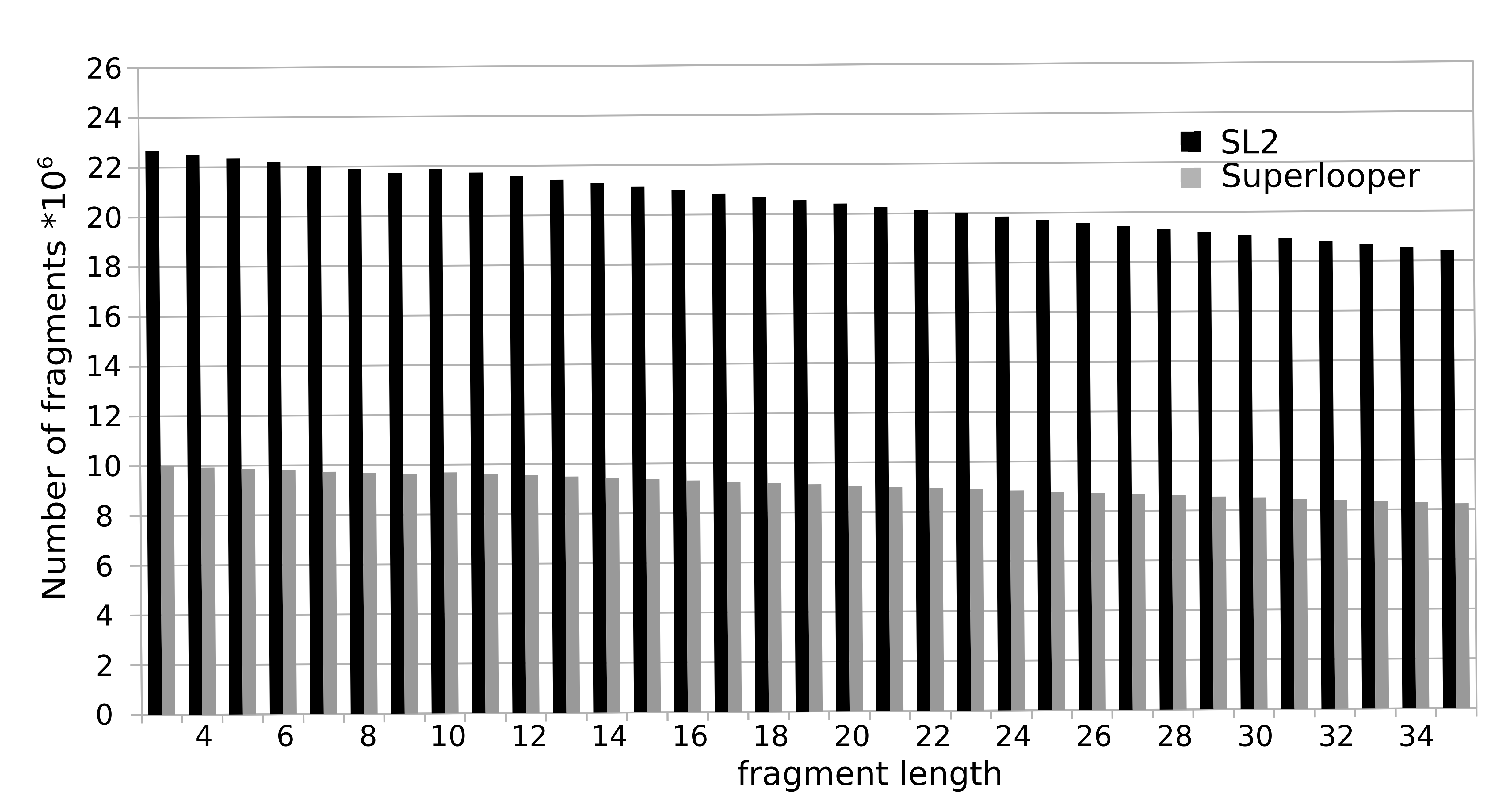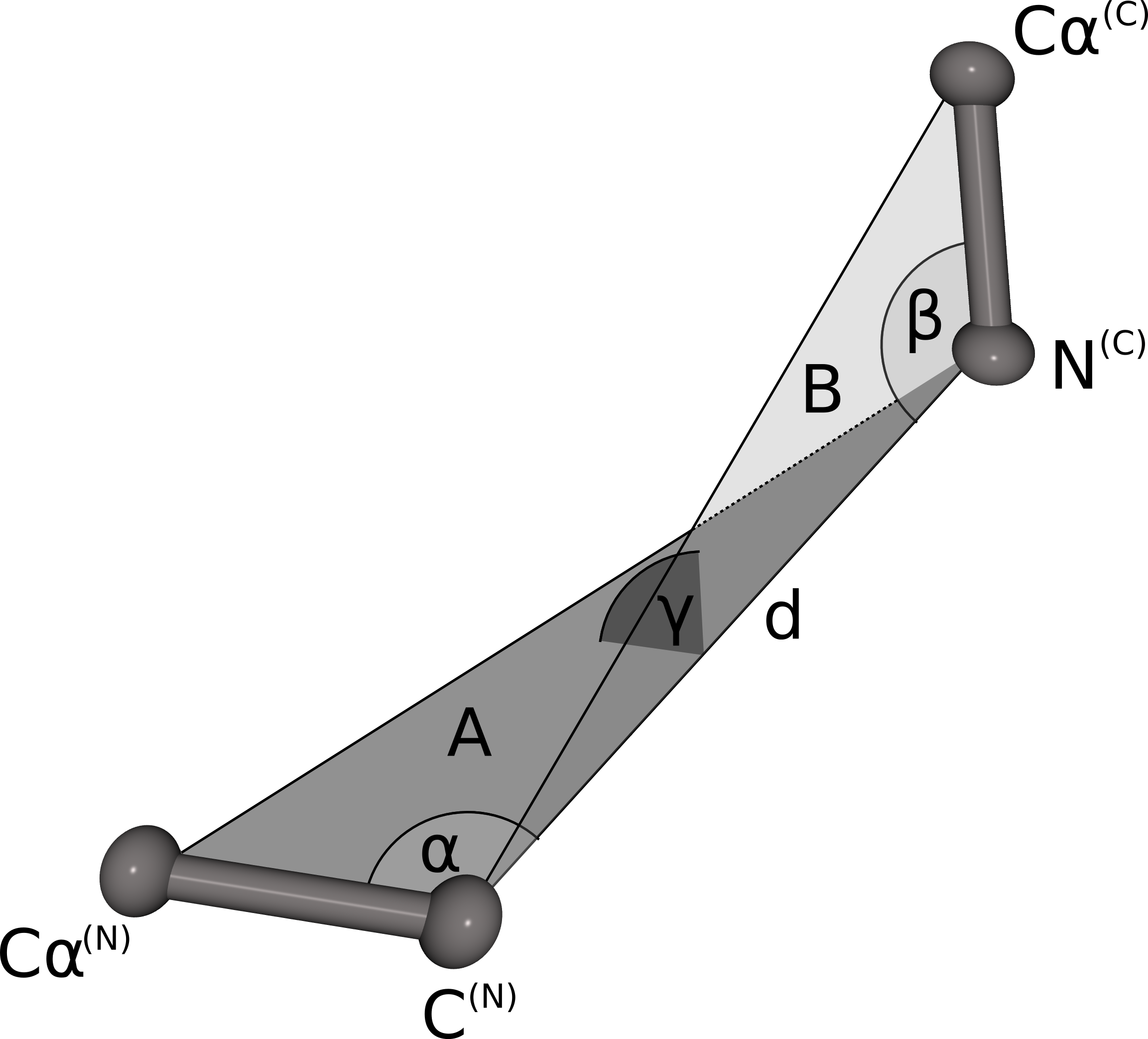# Methods

## Database

To construct the database all overlapping fragments with a length of 3 to 35 were extracted from all protein structures currently deposited in the PDB. For each fragment the following information is stored in the database: Sequence, PDB identifier, location in the protein and a geometrical fingerprint (see below). The total number of fragments sum up to over 1.000 million (Nov 2017). The number of available fragments is decreasing with increasing number of residues. This is caused by the fact that a smaller number of long fragments can be extracted from a structure, e.g. for the fragment length of 3 amino acids 23 million fragments are available, while for a fragment length of 35 this number declines to approximately 18 million.A second database holds fragments from helical membrane proteins. The number of helical transmembrane proteins in the PDB rose from 805 to >2.298 (Nov 2017). The number of loops in the database increased from 180.000 to >390.000 (Nov 2017). This database is also included in the default.

## Geometric fingerprint

The geometrical fingerprint matching is used to evaluate the sterical fit of the stem atoms of the N- and C-termini of each database fragment to the C- and N-terminal stem atoms of a gap in a protein structure. Both geometrical fingerprints are composed by the distance between the N- and C-terminal stem atoms and three angles defining the relative orientation of the stem atoms. The geometrical fingerprint is characterized by the distance d between the N-terminal C atom and the C-terminal N atom and the following three angles: α defined by the line between Cα(N) and C(N) and d, β is spanned by the line between N(C) and Cα(C) and d, γ defines the angle between the two planes A (defined by Cα(N), C(N)) and N(C)) and B (Cα(C), C(N) and N(C)). This fingerprint is a slight alteration of the fingerprint used in our previous publication. Instead of combining two distances and two angles we now use one distance and three angles. Analysis of the previous fingerprint revealed that the score was slightly biased towards the residue where angle was measured. By using angles on both stem residues, the resulting score does not favor the fit of the candidate fragment to one stem residue over the other any more.## Score

The 500 best loop candidates are ranked according to a score that is composed by a measure for the sequence identity and the fit of the stem atoms of loop and gap.

score = M - 0.1 * RMSD_stem2

The sequence score M is calculated using an environment-specific amino acid substitution matrix for accessible residues. The stem score RMSD is the root mean square deviation of the stem atoms Cα and C of the N-terminal stem residue and N and Cα of the C-terminal stem residues.

## Fragment Search

To minimize the calculation time of the fragment search a stepwise approach is used. In a first step, all fragments with the defined sequence length and which stem atoms matching the stem atoms of the gap with at least 0.75 Å RMSD are selected. In the second step, the 100 top candidates are chosen based on a quick estimation of the steric fit of the fragment to the rest of the protein e.g. excluding clashes. Subsequently, these 100 fragment are ranked by a score calculated from the sequence similarity and matching of the geometrical fingerprints of the (template) fragment and the target segment. To maximize the conformational space, fragments with an identical sequence or a backbone RMSD smaller than 1 Å are removed from the results list.

To minimize computation time, a minimal box containing the density of the missing fragment is extracted from the experimental map. The box size of the cryo-EM density map is reduced during two preprocessing steps. First, the box size is estimated from the maximum fragment length (i.e. assuming an unstructured conformation with maximal extend) and the distance between its stem atoms ('latitude'). A box is then cut out from the experimental EM-map with an edge length that doubles this latitude value and with its center at the midpoint between the two stem- atoms. Second, to prevent taking into account densities already occupied by atoms, these densities are deleted from the box prior calculation of cross correlation. For that purpose, existing structures within the minimal box are first converted into artificial densities with the intensity levels adjusted to the values of the experimental map. With a simple arithmetic operation, the artificial map is subtracted from the experimental map. These preprocessing steps therefore not only improve calculation time, but also performance. Finally the found fitting fragments are re-scored according to their cross-correlation to this preprocessed cryo-EM map.## Membrane planes

For the calculation of the membrane planes we employ the web-service TMDET. As result of the calculation TMDET  provides a list of residues that tangent the membrane. From the coordinates of Cα atoms of these residues, we then calculate a best-fit plane which is displayed in NGL.